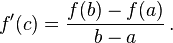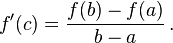# Mean Value Theorem

npellegrino
1. Use the Mean Value Theorem
f(x) = sqrtX - 2x at [0,4] so a = 0, b = 4

3. So I found the derivative (which is the slope) and then set the derivative equal to the 1 because of f(b)-f(a)/b-a
f'(x) = 1/2sqrtX - 2 so 1/2sqrtX - 2 = 0, then 1/2sqrtX = 2, then my solution is x = 1/16

Just would like a heads up if this is correct, thanks in advanced.

Mentor
1. Use the Mean Value Theorem
f(x) = sqrtX - 2x at [0,4] so a = 0, b = 4

3. So I found the derivative (which is the slope) and then set the derivative equal to the 1 because of f(b)-f(a)/b-a
f'(x) = 1/2sqrtX - 2 so 1/2sqrtX - 2 = 0, then 1/2sqrtX = 2, then my solution is x = 1/16

Just would like a heads up if this is correct, thanks in advanced.

No, this isn't correct. I think you probably made a mistake in your calculation of (f(b) - f(a))/(b - a), so check your work on this calculation.

Notice also that the extra parentheses I used (and you didn't) are necessary. The correct interpretation of what you wrote would be
$$f(b) - \frac{f(a)}{b} - a$$

and I'm sure that's not what you intended.

npellegrino
well the Mean Value Theorem isso if a = 0 and b = 4 wouldn't the solution be 1 ? then you set the derivative of the function to 1 which gave me 1/16 ?

Mentor
I think you might be calculating f'(a) and f'(b), instead of f(a) and f(b). Anyway (f(b) - f(a))/(b - a) $\neq$ 1.

cheddacheeze
subsitute the values of a and b into the formula
find the derivative of f(x)
and after youve got f'(x) and (f(b)-f(a))/b-a

npellegrino
the derivative i got for f(x) is 1/2sqrtX - 2 then i set that equals to (f(b)-f(a))/b-a which is 1, so i'm trying to figure 1/2sqrtX - 2 = 1 and i'm having trouble figuring the fraction

cheddacheeze
how are you getting the slope = 1, i got -6/4
f(4) = 4^1/2 - 8 = -6
f(0) = 0
(-6-0)/(4-0) does not equal to 1

well using my values i get 1/(2(x^1/2)) = -6/4+2
you can probably work your way from there

Mentor
the derivative i got for f(x) is 1/2sqrtX - 2 then i set that equals to (f(b)-f(a))/b-a which is 1, so i'm trying to figure 1/2sqrtX - 2 = 1 and i'm having trouble figuring the fraction
Stop already! (f(b)-f(a))/(b-a) IS NOT EQUAL TO 1 !

Put in the actual numbers for a and b and do the calculation!

npellegrino
You are not taking the derivatives of f(b) and f(a)... the mean value theorem states that if f is continuous on the closed intervals [a,b] which in this case is [0,4] and differentiable on the open interval (a,b,) then there exists a number c in (a,b) such thatnpellegrino
Stop already! (f(b)-f(a))/(b-a) IS NOT EQUAL TO 1 !

Put in the actual numbers for a and b and do the calculation!

maybe my professor has taught me wrong?

cheddacheeze
just look at the formula and substitute a and b... into the equation

npellegrino
Alright i'm having a brainfart i see what you are saying.

npellegrino
f(4) = sqrt4 - 8 = -6
f(0) = sqrt0 - 2(0) = 0

npellegrino
Alright i think i solved it... finally thanks for the help i really do appreciate it from you 2 :)

f(4) = -6
f(0) = 0
which gives me -6/4

the derivative 1/2sqrtX -2 = -6/4 which will give me 1/2sqrtx = 1/2 then x = 1

Mentor
Yes.

Mentor
maybe my professor has taught me wrong?
I'm pretty sure he didn't teach you that (2 - 8)/(4 - 0) = 1.

cheddacheeze
I'm pretty sure he didn't teach you that (2 - 8)/(4 - 0) = 1.

not so sure about that, sometimes the things they teach you are so obstract that they themselves get confusedMentor
No question that an instructor can occasionally get confused with some abstract calculation, but (2 - 8)/(4 - 0) in no way can be considered as abstract.

cheddacheeze
indeed it is not abstract, the mean value theorem is very elementary since its dealing with slopes and derivatives so its easy to understand